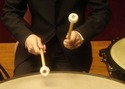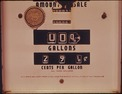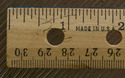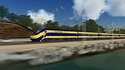## Rate

Type of Unit: Concept

# Prior Knowledge

Students should be able to:

• Solve problems involving all four operations with rational numbers.
• Understand quantity as a number used with a unit of measurement.
• Solve problems involving quantities such as distances, intervals of time, liquid volumes, masses of objects, and money, and with the units of measurement for these quantities.
• Understand that a ratio is a comparison of two quantities.
• Write ratios for problem situations.
• Make and interpret tables, graphs, and diagrams.
• Write and solve equations to represent problem situations.

# Lesson Flow

In this unit, students will explore the concept of rate in a variety of contexts: beats per minute, unit prices, fuel efficiency of a car, population density, speed, and conversion factors. Students will write and refine their own definition for rate and then use it to recognize rates in different situations. Students will learn that every rate is paired with an inverse rate that is a measure of the same relationship. Students will figure out the logic of how units are used with rates. Then students will represent quantitative relationships involving rates, using tables, graphs, double number lines, and formulas, and they will see how to create one such representation when given another.

Subject:
Mathematics, Algebra
Level:
Middle School
6
Tags:
• Algebra
• Equations
• Rate
• Unit 6 Rate•••••••••••••••